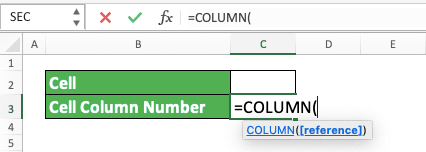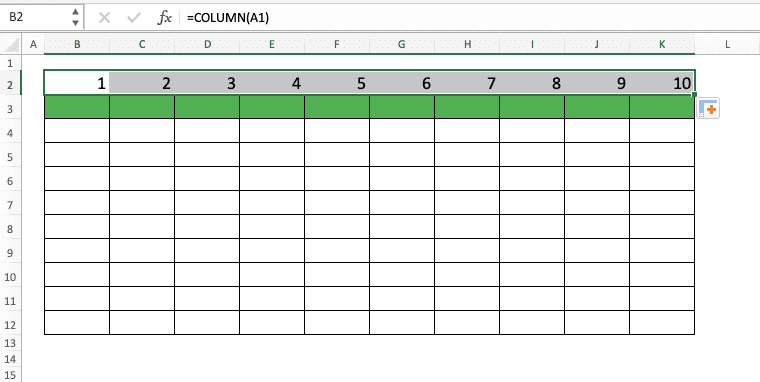How to Use the COLUMN Formula in Excel: Functions, Examples, and Writing Steps - Compute Expert

# How to Use the COLUMN Formula in Excel: Functions, Examples, and Writing Steps

Home >> Excel Tutorials from Compute Expert >> Excel Formulas List >> How to Use the COLUMN Formula in Excel: Functions, Examples, and Writing Steps

In this tutorial, you will learn how to use the COLUMN formula in excel completely.

When working in excel, we sometimes need to get the column number of a cell or a cell range. We might need to use that number for our data processing process or some other purposes. If we use the COLUMN formula in excel, then we can get that number fast and easily.

Want to know more about COLUMN and master the way to use this function properly in excel? Read this tutorial until its last part!

Disclaimer: This post may contain affiliate links from which we earn commission from qualifying purchases/actions at no additional cost for you. Learn more

## What is the COLUMN Formula in Excel?

COLUMN is an excel function that helps you to get the column number of a cell or a cell range.

## COLUMN Function in Excel

You can use COLUMN to get a cell or a cell range column number fast and easily in excel.

## COLUMN Result

The COLUMN result is a number that represents the column number of the cell or cell range you input into it.

## Excel Version from Which We Can Start Using COLUMN

We can start using COLUMN in excel 2003.

## The Way to Write It and Its Inputs

Here is the general writing form of the COLUMN function in excel.

= COLUMN ( [ cell_or_cell_range ] )

COLUMN only needs you to input the cell or cell range you want to get your column number from. This input is optional. If you don’t give any input to COLUMN, it will get the column number of the cell where you write it.

If you input a cell range, you will get the column number of the most left column in the cell range. If you want to get all column numbers in the cell range, however, you need to use an array formula form.

To use an array formula form, press Ctrl + Shift + Enter (instead of just Enter) buttons when you finish writing your COLUMN formula. You need to also highlight the cell range where you want to put your results (the cell range must have the same columns as the cell range you input) to get the numbers in full.

## Example of Its Usage and Result

Here is an implementation example of COLUMN in excel.In the example, you can see various COLUMN formula writings and the results we get from them.

When we don’t input anything to our COLUMN, it will simply return the column number of its cell. When we input a cell, however, it will return the column number of that cell.

When we input a cell range to COLUMN, it will return the most left column number in the cell range. That is unless we use the array formula and highlight a cell range with the same number of columns. We can get all the column numbers of our cell range that way.

## Writing Steps

Want guidance when you write a COLUMN formula in excel? Use the COLUMN formula writing steps we have written for you below!

1. Type an equal sign ( = ) in the cell where you want to put your COLUMN result2. Type COLUMN (can be with large and small letters) and an open bracket sign after =3. Optional: Input the cell or cell range you want to get your column number from. If you want all column numbers from your cell range, then do this first before you write your COLUMN formula.

Highlight a cell range with the same number of columns as your cell range input. This is where COLUMN will place the cell range column numbers.

If you don’t input anything, COLUMN will get the column number of the cell where you write it4. Type a close bracket sign5. Press Enter (or press Ctrl + Shift + Enter if you input a cell range and want to get all its column numbers)
6. Done!## Exercise

### Questions

Use COLUMN to answer each question below in the appropriate gray-colored cell!
1. What is the most left column number of the yellow cell range?
2. What is the column number of the gray cell?
3. What are the numbers of all the columns in the blue cell range? Place your answers by using the gray cell as the most left cell of the answers!

By using COLUMN, you can create a numbering for your data table columns in excel easily.

Just write the COLUMN formula for the most left column number of the data table. Then, use the excel autofill feature to copy it until the most right column of your data table.Related tutorials you should learn from:

Get updated excel info from Compute Expert by registering your email. It's free!